# 1606 A Lucky Shamrock

### Today’s Puzzle:

Even if you don’t know all the factors of some of these clues in this shamrock puzzle, there are enough that you will know, and you can figure the rest out easily. Lucky you!Print the puzzles or type the solution in this excel file: 14 Factors 1604-1612.

### Factors of 1606:

• 1606 is a composite number.
• Prime factorization: 1606 = 2 × 11 × 73.
• 1606 has no exponents greater than 1 in its prime factorization, so √1606 cannot be simplified.
• The exponents in the prime factorization are 1, 1, and 1. Adding one to each exponent and multiplying we get (1 + 1)(1 + 1)(1 + 1) = 2 × 2 × 2 = 8. Therefore 1606 has exactly 8 factors.
• The factors of 1606 are outlined with their factor pair partners in the graphic below.### More about the Number 1606:

1606 is the hypotenuse of a Pythagorean triple:
1056-1210-1606. which is 22 times (48-55-73).

1606 is in a couple of other Pythagorean triples that can be calculated from
2(803)(1), 803² – 1², 803² + 1², and
2(73)(11), 73² – 11², 73² + 11².

# 1595 and Level 2

### Today’s Puzzle:

This puzzle is just a multiplication table whose missing factors are not in the usual order. Can you figure out where the factors from 1 to 10 should go?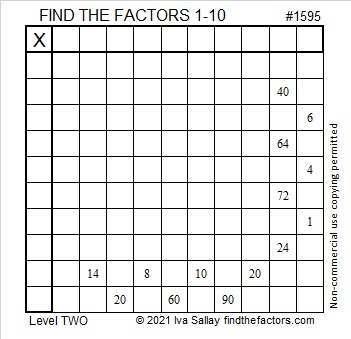### Factors of 1595:

1595 ends with a 5, so it is divisible by 5.
1 – 5 + 9 – 5 = 0, so 1595 is divisible by 11.

• 1595 is a composite number.
• Prime factorization: 1595 = 5 × 11 × 29.
• 1595 has no exponents greater than 1 in its prime factorization, so √1595 cannot be simplified.
• The exponents in the prime factorization are 1, 1, and 1. Adding one to each exponent and multiplying we get (1 + 1)(1 + 1)(1 + 1) = 2 × 2 × 2 = 8. Therefore 1595 has exactly 8 factors.
• The factors of 1595 are outlined with their factor pair partners in the graphic below.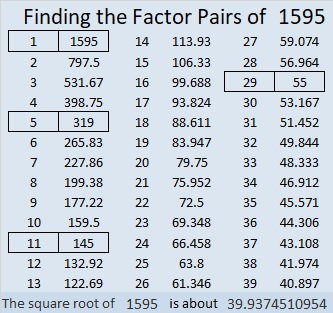### More about the Number 1595:

1595 is the hypotenuse of FOUR Pythagorean triples:
187-1584-1595, which is 11 times (17-144-145),
264-1573-1595, which is 11 times (24-143-145),
957-1276-1595, which is (3-4-5) times 319, and
1100-1155-1595, which is (20-21-29) times 55.

# 1587 XOXOXO Hugs and Kisses OXOXOX

### Today’s Puzzle:

Hugs are often abbreviated as “O” in letters and notes, and kisses are abbreviated as “X”. This puzzle can be a Valentine’s card and will send hugs and kisses to whomever you give it.

Can you write the numbers from 1 to 12 in both the first column and the top row so that those numbers and the given clues function like a multiplication table?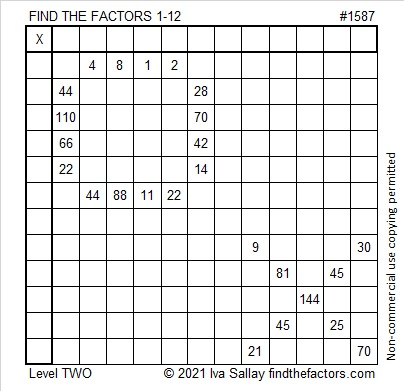### Factors of 1587:

1587 is divisible by 3 because 1 + 5 + 8 + 7 = 21, a number divisible by 3.
1587 ÷ 3 = 529. Perhaps you have memorized some perfect squares and remember that 23² = 529. (If your parents invest in a 529 college plan for your education, you are more likely to have a college degree by the time you are 23. That’s how I remember it!)

• 1587 is a composite number.
• Prime factorization: 1587 = 3 × 23 × 23, which can be written 1587 = 3 × 23².
• 1587 has at least one exponent greater than 1 in its prime factorization so √1587 can be simplified. Taking the factor pair from the factor pair table below with the largest square number factor, we get √1587 = (√529)(√3) = 23√3.
• The exponents in the prime factorization are 1 and 2. Adding one to each exponent and multiplying we get (1 + 1)(2 + 1) = 2 × 3 = 6. Therefore 1587 has exactly 6 factors.
• The factors of 1587 are outlined with their factor pair partners in the graphic below.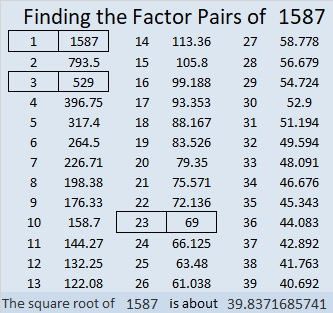### More about the Number 1587:

1587 is the difference of two squares three different ways:
794² – 793² = 1587,
266² – 263² = 1587, and
46² – 23² = 1587.

# 1577 Crossing a Bridge

### Today’s Puzzle:

Merriam-Webster defines a bridge as “a structure carrying a pathway or roadway over a depression or obstacle (such as a river)”. Bridges have been built over wide rivers, but what type of bridge is needed when the obstacle is prejudice, hate, or greed?

Today is Martin Luther King Jr. Day in the United States. He tirelessly worked on such bridges. The movie, Selma, portrays much of what he and his companions did to cross a certain bridge in Alabama.  Although ALL of his protests were peaceful, some who followed him were brutally killed. Dr. King himself was assassinated when he was just 39 years old.

Today bridges still need to be built. Education can be an important bridge-builder. If you have Netflix, type “Martin Luther King” into the search, and several eye-opening movies and documentaries will appear. I highly recommend 13th, a documentary that shows how the same amendment to the constitution that abolished slavery has a loophole that essentially allows slavery to continue to this day. I was appalled.

The puzzle below is easy to solve. How to build bridges that make the world better for EVERYONE is a far more important and difficult puzzle that each of us needs to ponder to get closer to a solution.### Factors of 1577:

• 1577 is a composite number.
• Prime factorization: 1577 = 19 × 83.
• 1577 has no exponents greater than 1 in its prime factorization, so √1577 cannot be simplified.
• The exponents in the prime factorization are 1 and 1. Adding one to each exponent and multiplying we get (1 + 1)(1 + 1) = 2 × 2 = 4. Therefore 1577 has exactly 4 factors.
• The factors of 1577 are outlined with their factor pair partners in the graphic below.### More about the Number 1577:

1577 is the difference of two squares two different ways:
789² – 788² = 1577, and
51² – 32² = 1577.

1577 is the sum of the 2 numbers from 788 to 789.
1577 is the sum of the 19 numbers from 74 to 92. (83 is in the exact middle of that list of numbers.)
1577 is the sum of the 38 numbers from 23 to 60.

# 1549 and Level 2

### Today’s Puzzle:

Write the numbers from 1 to 10 in both the first column and the top row so that the given clues are the products of the numbers you write.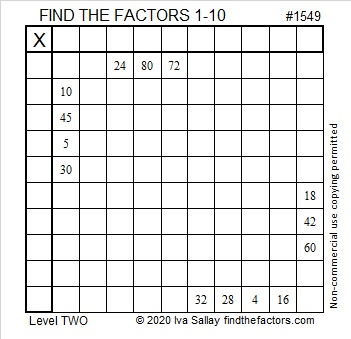### Factors of 1549:

• 1549 is a prime number.
• Prime factorization: 1549 is prime.
• 1549 has no exponents greater than 1 in its prime factorization, so √1549 cannot be simplified.
• The exponent in the prime factorization is 1. Adding one to that exponent we get (1 + 1) = 2. Therefore 1549 has exactly 2 factors.
• The factors of 1549 are outlined with their factor pair partners in the graphic below.How do we know that 1549 is a prime number? If 1549 were not a prime number, then it would be divisible by at least one prime number less than or equal to √1549. Since 1549 cannot be divided evenly by 2, 3, 5, 7, 11, 13, 17, 19, 23, 29, 31, or 37, we know that 1549 is a prime number.

### More about the number 1549:

1549 is the sum of two squares:
35² + 18² = 1549

1549 is the hypotenuse of a Pythagorean triple:
901-1260-1549, calculated from 35² – 18², 2(35)(18), 35² + 18².

Here’s another way we know that 1549 is a prime number: Since its last two digits divided by 4 leave a remainder of 1, and 35² + 18² = 1549 with 35 and 18 having no common prime factors, 1549 will be prime unless it is divisible by a prime number Pythagorean triple hypotenuse less than or equal to √1549. Since 1549 is not divisible by 5, 13, 17, 29, or 37, we know that 1549 is a prime number.

# 1543 Another Letter

### Today’s Puzzle:

My goal was to make this next letter of my message using as few clues in the puzzle as possible. You now have enough clues to solve this puzzle and know my message to you.### Factors of 1543:

• 1543 is a prime number.
• Prime factorization: 1543 is prime.
• 1543 has no exponents greater than 1 in its prime factorization, so √1543 cannot be simplified.
• The exponent in the prime factorization is 1. Adding one to that exponent we get (1 + 1) = 2. Therefore 1543 has exactly 2 factors.
• The factors of 1543 are outlined with their factor pair partners in the graphic below.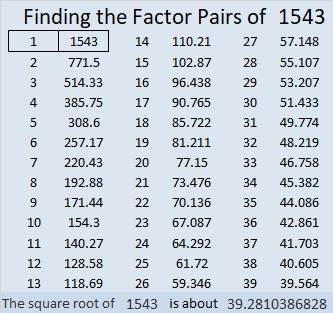How do we know that 1543 is a prime number? If 1543 were not a prime number, then it would be divisible by at least one prime number less than or equal to √1543. Since 1543 cannot be divided evenly by 2, 3, 5, 7, 11, 13, 17, 19, 23, 29, 31, or 37, we know that 1543 is a prime number.

### One More Fact about the Number 1543:

1543 is the sum of consecutive numbers as well as the difference of those same consecutive numbers but squared:
771 + 772 = 1543;
772² – 771² = 1543.
(1543 has that property because it’s an odd number greater than 1.)

# 1537 First Letter of a Secret Message

### Today’s Puzzle:

The next six puzzles will look like letters of the alphabet, and they will spell out a secret message for you. Will you figure out the message before I have published all of the letters? The difficulty levels of the puzzles will not be in any particular order.### Factors of 1537:

OEIS.org tells us that the largest prime factor of 1537 is 53. Pretty cool!

• 1537 is a composite number.
• Prime factorization: 1537 = 29 × 53.
• 1537 has no exponents greater than 1 in its prime factorization, so √1537 cannot be simplified.
• The exponents in the prime factorization are 1 and 1. Adding one to each exponent and multiplying we get (1 + 1)(1 + 1) = 2 × 2 = 4. Therefore 1537 has exactly 4 factors.
• The factors of 1537 are outlined with their factor pair partners in the graphic below.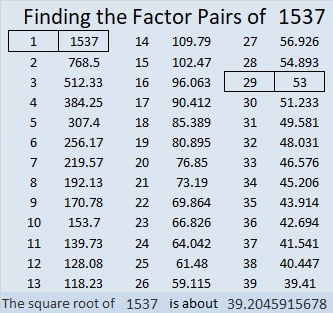### More about the Number 1537:

1537 is the DIFFERENCE of two squares in two different ways:
769² – 768² = 1537, and
41² – 12² = 1537.

1537 is also the SUM of two squares in two different ways:
39² + 4² = 1537, and
31² + 24² = 1537.

1537 is the hypotenuse of FOUR Pythagorean triples:
312-1505-1537, calculated from 2(39)(4), 39² – 4², 39² + 4²,
385-1488-1537, calculated from 31² – 24², 2(31)(24), 31² + 24²,
812-1305-1537, which is 29 times (28-45-53), and
1060-1113-1537, which is (20-21-29) times 53.

# 1527 Not a Haunted House

### Today’s Puzzle:

We see plenty of pumpkins on doorsteps this time of year, so I put a few in this puzzle. The puzzle looks a bit like a house, but certainly not a haunted house. Can you write the numbers from 1 to 10 in both the first column and the top row so that the given number clues are the products of those numbers?

Here’s the same puzzle without the added colorful embellishments:### Factors of 1527:

• 1527 is a composite number.
• Prime factorization: 1527 = 3 × 509.
• 1527 has no exponents greater than 1 in its prime factorization, so √1527 cannot be simplified.
• The exponents in the prime factorization are 1, and 1. Adding one to each exponent and multiplying we get (1 + 1)(1 + 1) = 2 × 2 = 4. Therefore 1527 has exactly 4 factors.
• The factors of 1527 are outlined with their factor pair partners in the graphic below.### Another Fact about the Number 1527:

1527 is the hypotenuse of a Pythagorean triple:
660-1377-1527, which is 3 times (220-459-509).

# 1514 and Level 2

### Today’s Puzzle:

There is only one way to write the factors from 1 to 12 in both the first column and the top row so that this puzzle will behave like a multiplication table. The given clues will be the products of the factors you write. Can you find the way?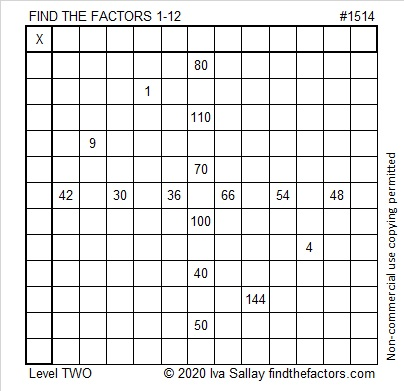### Factors of 1514:

• 1514 is a composite number.
• Prime factorization: 1514 = 2 × 757.
• 1514 has no exponents greater than 1 in its prime factorization, so √1514 cannot be simplified.
• The exponents in the prime factorization are 1, and 1. Adding one to each exponent and multiplying we get (1 + 1)(1 + 1) = 2 × 2 = 4. Therefore 1514 has exactly 4 factors.
• The factors of 1514 are outlined with their factor pair partners in the graphic below.### More Facts about the number 1514:

1514 is the sum of two squares:
35² + 17² = 1514

1514 is the hypotenuse of a Pythagorean triple:
936-1190-1514 calculated from 35² – 17², 2(35)(17), 35² + 17²

# Why is 1503 a Friedman Number?

### Friedman Puzzle:

Can you find an expression equaling 1503 that uses 1, 5, 0, and 3 each exactly once, but in any order, and some combination of  +, -, ×, or ÷? For this particular Friedman puzzle, none of those digits are exponents. If you can solve this Friedman puzzle, you will know why 1503 is the 24th Friedman number. You can find the solution hidden someplace in this post. (By the way, another permutation of those digits, 1530, will be the 25th Friedman number!)

### Find the Factors Puzzle:

There are 14 clues in this level 2 puzzle. Use those clues and logic to place the factors 1 to 10 in both the first column and the top row. That’s how you start to turn this puzzle into a multiplication table!### Factors of 1503:

• 1503 is a composite number.
• Prime factorization: 1503 = 3 × 3 × 167, which can be written 1503 = 3² × 167.
• 1503 has at least one exponent greater than 1 in its prime factorization so √1503 can be simplified. Taking the factor pair from the factor pair table below with the largest square number factor, we get √1503 = (√9)(√167) = 3√167.
• The exponents in the prime factorization are 2 and 1. Adding one to each exponent and multiplying we get (2 + 1)(1 + 1) = 3 × 2 = 6. Therefore 1503 has exactly 6 factors.
• The factors of 1503 are outlined with their factor pair partners in the graphic below.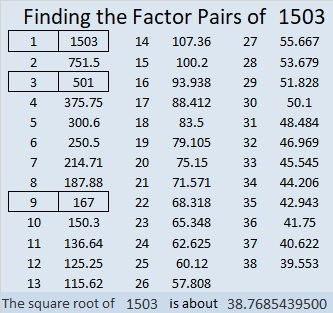Did you see the solution to the Friedman puzzle in that factor pair chart?